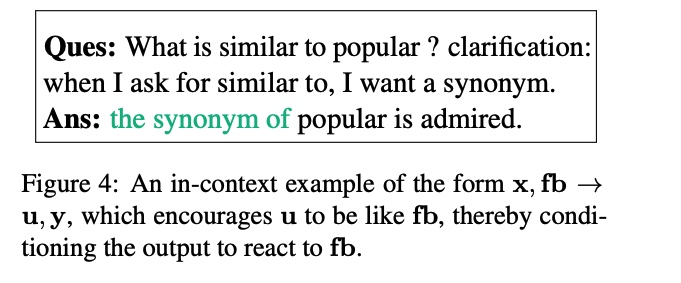MemPrompt is a general technique that can be used to patch GPT-3 after deployment. To adapt MemPrompt for a task, we need to create prompts that include the following two components: (i) generating an understanding of the task ($u$), and (ii) reacting to user feedback ($fb$). Please see Section 3 of the paper for more details.

## Generating Task Understanding with Response

Existing methods for receiving user feedback typically assume the user knows the correct answer. This assumption is paradoxical: if the user knew the answer, why would they be using the model?

In real settings, the user may not know the correct answer, but may know what instruction they gave. Thus, MemPrompt generates task understanding in response in addition to the answer. We operationalize this idea by including task verbalization in the prompt.

Given a question ($x$) What sounds like < sighted > ?, a simple prompting approach will generate the answer ($y$) cited (say). In contrast, we prompt the model to generate a task description the homophone for.

To see why this is helpful, consider a test question What sounds similar to < sighted > ?. If the model generates the word that has the same meaning as its understanding of task, the user has a reason to believe that the answer is wrong.## Allowing GPT-3 to react to feedback

Once the feedback is received from the user, can the model successfully utilize it? By adding a few examples of the form $x, fb \rightarrow u, y$ in the prompt and setting $fb=u$, we force the model to use the task understanding present in the input when generating the output~(Figure below).